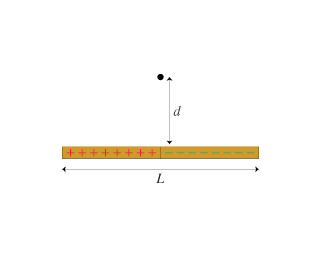# Problem: The two halves of the rod in the figure are uniformly charged to plus/minus Q as shown.What is the electric potential at the point indicated by the dot?

###### FREE Expert Solution

The point is equidistant from the two halves. The charge in the two halves also has equal magnitude.

97% (275 ratings)###### Problem Details

The two halves of the rod in the figure are uniformly charged to plus/minus Q as shown.

What is the electric potential at the point indicated by the dot?